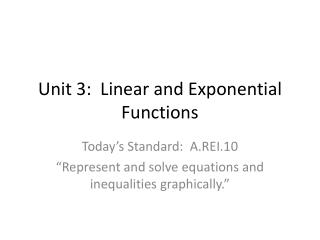# Unit 3: Linear and Exponential Functions - PowerPoint PPT PresentationDownload PresentationUnit 3: Linear and Exponential Functions

Unit 3: Linear and Exponential FunctionsDownload Presentation## Unit 3: Linear and Exponential Functions

- - - - - - - - - - - - - - - - - - - - - - - - - - - E N D - - - - - - - - - - - - - - - - - - - - - - - - - - -
##### Presentation Transcript

1. Unit 3: Linear and Exponential Functions Today’s Standard: A.REI.10 “Represent and solve equations and inequalities graphically.”

2. Essential Question: • How do I make a table of values and plot the points on a coordinate plane for linear and exponential functions?

3. Language of the Standard MCC9-12.A.REI.10: Understand that the graph of an equation in two variables is the set of all its solutions plotted in the coordinate plane, often forming a line or a curve.

4. What we will be doing today: • Basically you are going to be given a function, (either linear or exponential), and asked to make a table of values, then plot the points on a coordinate plane, and draw a line/curve.

5. Steps: • Assign these values for x: -2, -1, 0, 1, and 2 • Solve for y by plugging each value in for x and working the problem out (you will be dealing with fractions so GET EXCITED!!) • Plot the points on a coordinate plane • Draw your line or curve **You will need to remember your negative exponent rules when solving an exponential function

6. Example 1 y = 2x - 1

7. Example 2: 3x + 2y = 6

8. Example 3: y = 2• 3x

9. Example 4: y = ½ • (¼)x

10. Assignment: • worksheet